# Overload (多載) vs. Override (覆寫) — (I)

Overload (多載)Override (覆寫) 為程式設計的 2 個常見性質，

「多個相同方法名稱」、「改寫父類別方法」…，而不懂實際運用。[註]: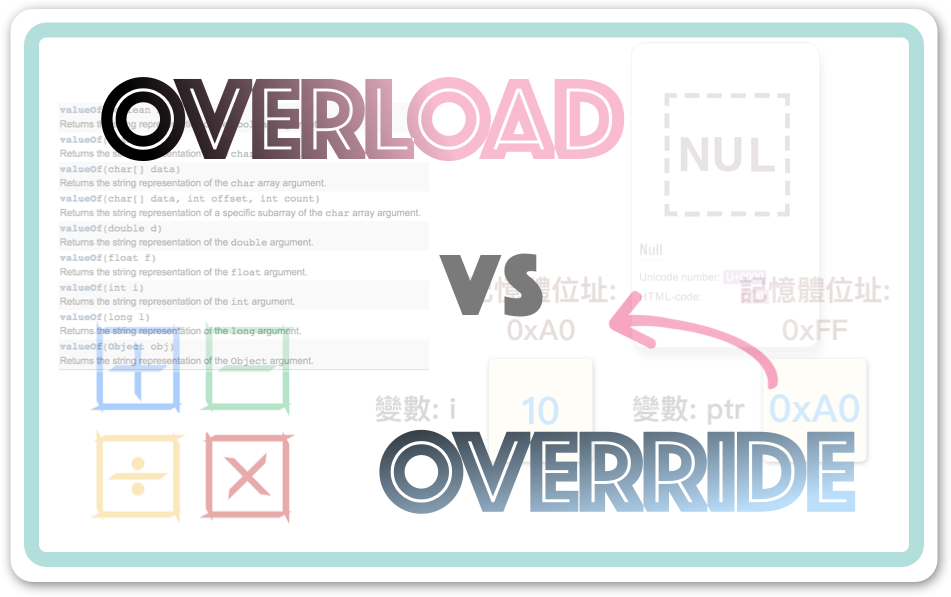### 其實你學過了！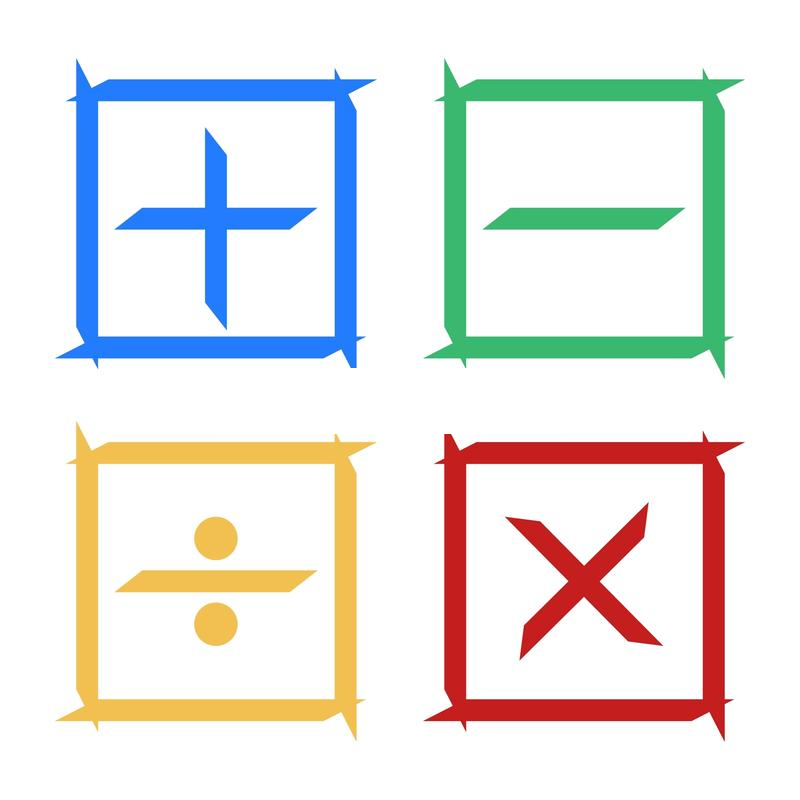— — 將多載觀念：『相同的模樣，擁有不同的行為』，應用在運算子上。

``````public class Main {

public static void main(String[] args) {

String name = "Jason";
String output = "Hello, " + name + "!";

System.out.println(output);
}
}

/*
* Output:
*
* Hello, Jason!
*/``````

[註]:

Java、C… 並不 提供『自訂的運算子多載』，詳見 此篇

( 包含不同的參數型別、數量 )

``````void test();
void test(int i);
void test(char c);
void test(String s, int i);
void test(String s, String s2);``````

[註]:

#### 無多載方法

Bob 是個愛煎牛排的固執廚師 (chef)：``````public class Main {

public static void main(String[] args) {

Chef bob = new Chef();

bob.cook(); // 牛排x1
}
}

// 廚師 (chef)
class Chef {

// 回傳型別： void
// 參數型別： 無
void cook() {
System.out.println("準備餐點: 牛排");
}
}``````

#### 多載方法 (增加參數)

``````public class Main {

public static void main(String[] args) {

Chef bob = new Chef();

bob.cook(); // 牛排x1

bob.cook('B'); // 豬排x1

bob.cook('C'); // 食屎
}
}

class Chef {

// 回傳型別： void
// 參數型別： 無
void cook() {
System.out.println("準備餐點: 牛排");
}

// 回傳型態： void
// 參數型態： char
void cook(char meal) {
switch (meal) {
case 'A':
System.out.println("準備 A 號餐: 牛排");
break;
case 'B':
System.out.println("準備 B 號餐: 豬排");
break;
default:
System.out.println("食屎吧你");
break;
}
}
}``````

#### 多載方法 (多個參數)

``````// 回傳型別： void
// 參數型別： char, int
void cook(char meal, int quantity) {
if (quantity < 1)
return;
switch (meal) {
case 'A':
System.out.println("準備 A 號餐: 牛排");
break;
case 'B':
System.out.println("準備 B 號餐: 豬排");
break;
default:
System.out.println("食屎吧你");
break;
}
// 做好一份了，剩下 quantity - 1 份
cook(meal, quantity - 1); // 遞迴呼叫
}``````

[註]:

``````public class Main {

public static void main(String[] args) {

Chef bob = new Chef();

bob.cook('A'); // 牛排x1

bob.cook('B', 3); // 豬排x3
}
}``````

cook( )  vs.  cook(“A”)  vs.  cook(‘B’, 3);

#### 方法簽章 (Method Signature)

``````class Chef {

// 回傳型別： void
// 參數型別： 無
void cook() {
... 略 ...
}

// 回傳型別： void
// 參數型別： char
void cook(char meal) {
... 略 ...
}

// 回傳型別： void
// 參數型別： char, int
void cook(char meal, int quantity) {
... 略 ...
}
}``````

Q1: 請問，是否能再擴充以下方法？ (參數串列 與 `cook(char meal)` 衝突，但 修改回傳型態 )

``````boolean cook(char meal){
... 略 ...
}``````

Ans:

[註]:

Q2：請問，是否能再擴充以下方法？ (參數串列 與 Chef 類別 衝突，但 修改回傳型態 )

``````boolean cook(int i){
... 略 ...
}``````

Ans:

— — 軟體開發大師 Kent Beck.

#### 重構 — 重複的程式碼 (Duplicated Code)

``````class Chef {

void cook() {
System.out.println("準備 A 號餐: 牛排");
}

void cook(char meal) {
switch (meal) {
case 'A':
System.out.println("準備 A 號餐: 牛排");
break;
case 'B':
System.out.println("準備 B 號餐: 豬排");
break;
default:
System.out.println("食屎吧你");
break;
}
}

void cook(char meal, int quantity) {

if (quantity < 1)
return;

switch (meal) {
case 'A':
System.out.println("準備 A 號餐: 牛排");
break;
case 'B':
System.out.println("準備 B 號餐: 豬排");
break;
default:
System.out.println("食屎吧你");
break;
}

// 做好一份了，剩下 (quantity - 1) 份
cook(meal, quantity - 1); // 遞迴呼叫
}
}```````void cook()` 方法，能夠 點一份牛排
`void cook(char meal)` 方法，能 點一份牛排 或豬排，

``````void cook() {
cook('A'); // 呼叫多載函式
}

void cook(char meal) {
switch (meal) {
case 'A':
System.out.println("準備 A 號餐: 牛排");
break;
case 'B':
System.out.println("準備 B 號餐: 豬排");
break;
default:
System.out.println("食屎吧你");
break;
}
}``````

`void cook(char meal)` 只能 點一份 牛排或豬排，
`void cook(char meal, int quantity)` 能夠 點很多份 牛排或豬排！

``````void cook(char meal) {
cook(meal, 1); // 相當於數量 x1
}``````

``````class Chef {

// 點一份 牛排
void cook() {
cook('A');
}

// 點一份 牛排 or 豬排
void cook(char meal) {
cook(meal, 1);
}

// 點很多份 牛排 or 豬排
void cook(char meal, int quantity) {

if (quantity < 1)
return;

switch (meal) {
case 'A':
System.out.println("準備 A 號餐: 牛排");
break;
case 'B':
System.out.println("準備 B 號餐: 豬排");
break;
default:
System.out.println("食屎吧你");
break;
}

// 做好一份了，剩下 quantity - 1 份
cook(meal, quantity - 1); // 遞迴呼叫
}
}``````

But，得注意的是：

``````class Rectangle {

int width;
int height;
int color;

Rectangle() {
this.width = 500;
// 別問我為何 1.618...去問歐幾里得
this.height = (int) (width / 1.618);
this.color = 0x2196F3; // 藍色
}

Rectangle(int width) {
this.width = width;
this.height = (int) (width / 1.618);
this.color = 0x2196F3; // 藍色
}

Rectangle(int width, int height) {
this.width = width;
this.height = height;
this.color = 0x2196F3; // 藍色
}

Rectangle(int width, int height, int color) {
this.width = width;
this.height = height;
this.color = color;
}
}````````````class Rectangle {

int width;
int height;
int color;

Rectangle() {
this(500); // 正確 (O)
//        Rectangle(width); 錯誤 (X) -- 得使用 this 關鍵字
}

Rectangle(int width) {
this(width, (int) (width / 1.618));
}

Rectangle(int width, int height) {
//        int area = width * height; 錯誤 (X) -- 利用「this」呼叫建構子，需為首句敘述
this(width, height, 0x2196F3);
}

// 主建構函式
Rectangle(int width, int height, int color) {
this.width = width;
this.height = height;
this.color = color;
}
}``````

this() 和 this. 不一樣喔！
`this()` 用於 在 建構元中 呼叫 其他建構元，且需為 首句敘述 (first statement)，
`this.` 用於存取類別中的欄位 (例 this.width)，放第一萬行也沒關係！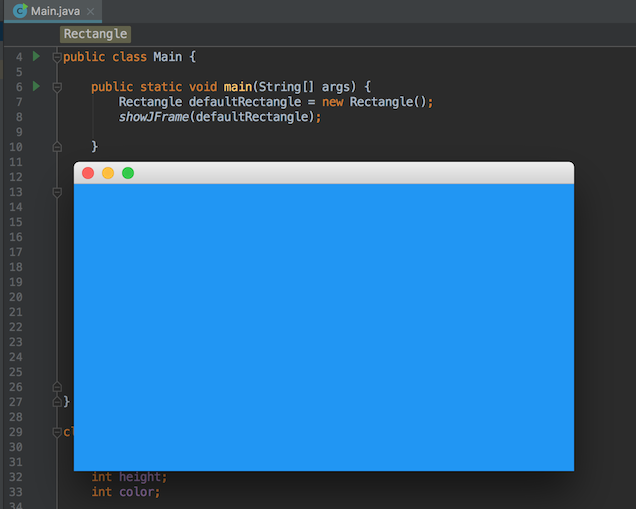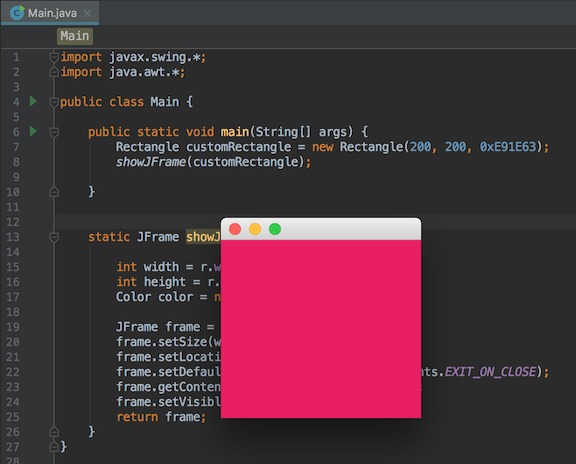``````import javax.swing.*;
import java.awt.*;

public class Main {

public static void main(String[] args) {
Rectangle defaultRec = new Rectangle();
showJFrame(defaultRec);
}

static JFrame showJFrame(Rectangle r) {

int width = r.width;
int height = r.height;
Color color = new Color(r.color);

// 簡易的 Swing 圖形化介面
JFrame frame = new JFrame();
frame.setSize(width, height);
frame.setLocationRelativeTo(null);
frame.setDefaultCloseOperation(WindowConstants.EXIT_ON_CLOSE);
frame.getContentPane().setBackground(color);
frame.setVisible(true);
return frame;
}
}``````

#### 子類別的多載

``````public class Main {

public static void main(String[] args) {
new A().test(); // A

new B().test(); // B

new B().test('C'); // C
}
}

class A {
void test() {
System.out.println('A');
}
}

class B extends A {

// 覆寫 (Override)
void test() {
System.out.println('B');
}

void test(char c) {
System.out.println(c);
}
}``````

( 包含不同的參數型別、數量 )

### 總結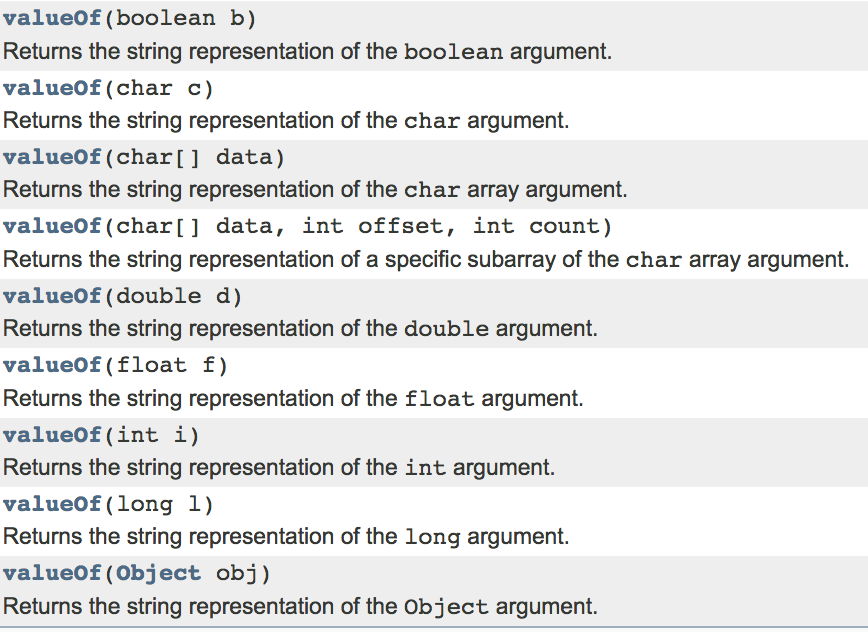Override (覆寫) 的觀念將在 下篇 提及 😁。#### 在《Overload (多載) vs. Override (覆寫) — (I)》中有 12 則留言

1.Sandra表示:

獲益良多，非常感謝，是個非常優秀的老師😁

2.吳建儒四資工三甲表示:

受益匪淺，感謝分享。

3.匿名訪客表示:

感謝大神

4.匿名訪客表示:

這個很有用,感謝～～～～

5.匿名訪客表示:

真的很實用!感謝您

6.匿名訪客表示:

解釋的詳細又清楚，謝謝你～

1.鄭 中勝表示:

謝謝你們 😃

7.zimo wang表示:

感谢分享，例子非常简单易懂

1.鄭 中勝表示:

謝謝 😄

1.匿名訪客表示:

謝謝！講的很詳細 :

1.鄭 中勝表示:

非常感謝！😆

8.匿名訪客表示:

例子淺顯易懂 講解得非常棒 謝謝您的說明!